Processing ......FreeComputerBooks.com Free Computer, Mathematics, Technical Books and Lecture Notes, etc.

Algebra, Abstract Algebra, and Linear Algebra, etc.Related Book Categories:
•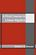A First Course in Linear Algebra (Robert A Beezer)

This book is an introduction to the basic concepts of linear algebra, along with an introduction to the techniques of formal mathematics. It begins with systems of equations and matrix algebra before moving into the advanced topics.

•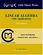Linear Algebra with Applications (W. Keith Nicholson)

The aim of the text is to achieve a balance among computational skills, theory, and applications of linear algebra. It is a relatively advanced introduction to the ideas and techniques of linear algebra targeted for science and engineering students.

•Applied Linear Algebra: Vectors, Matrices, and Least Squares

Combines straightforward explanations with a wealth of practical examples to offer an innovative approach to teaching linear algebra. Requiring no prior knowledge of the subject, it covers the aspects of linear algebra - vectors, matrices, and least squares

•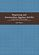Beginning and Intermediate Algebra (Tyler Wallace)

These books use a teacherly writing style and a careful blend of skills development and conceptual questions to meet the unique needs of the developmental math student. It takes advantage of experiences in the classroom and an editing eye to offer one of the most well-rounded series available, written with the developmental learner in mind.

•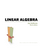Linear Algebra (Jim Hefferon)

This textbook covers linear systems and Gauss' method, vector spaces, linear maps and matrices, determinants, and eigenvectors and eigenvalues. Each chapter has three or four discussions of additional topics and applications.

•Linear Algebra: A Course for Physicists and Engineers (Arak Mathai)

This textbook on linear algebra is written to be easy to digest by non-mathematicians. It introduces the concepts of vector spaces and mappings between them without too much theorems and proofs. Various applications of the formal theory are discussed as well.

•Applied Linear Algebra in Action (Vasilios N. Katsikis)

This textbook contains a collection of six high-quality chapters. The techniques are illustrated by a wide sample of applications. This book is devoted to Linear Mathematics by presenting problems in Applied Linear Algebra of general or special interest.

•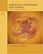Modeling, Functions, and Graphs: Algebra for College Students

This book includes content found in a typical algebra course, along with introductions to curve-fitting and display of data. The authors focus on three core themes throughout their textbook: Modeling, Functions, and Graphs.

•Elementary Abstract Algebra: Examples and Applications

It introduces the basic notions of group theory by a thorough treatment of important examples, including complex numbers, modular arithmetic, symmetries, and permutations, also applications to communications, cryptography, and coding theory.

•Advanced Algebra (Anthony W. Knapp)

This book is on modern algebra which treat various topics in commutative and noncommutative algebra and provide introductions to the theory of associative algebras, homological algebras, algebraic number theory, and algebraic geometry.

•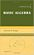Basic Algebra (Anthony W. Knapp)

This book is on Preliminaries about the Integers, Polynomials, and Matrices; Vector Spaces over Q, R, and C; Inner-Product Spaces; Groups and Group Actions; Theory of a Single Linear Transformation; Multilinear Algebra; Advanced Group Theory; etc.

•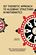Set Theoretic Approach to Algebraic Structures in Mathematics

This book brings out how sets in algebraic structures can be used to construct the most generalized algebraic structures, like set linear algebra / vector space, set ideals in groups and rings and semigroups, and topological set vector spaces.

•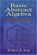Basic Abstract Algebra (Robert B. Ash)

This book surveys fundamental algebraic structures and maps between these structures. Its techniques are used in many areas of mathematics, with applications to physics, engineering, and computer science as well.

•Abstract Algebra: Theory and Applications (Thomas W Judson)

This book is designed to teach the principles and theory of abstract algebra to college juniors and seniors in a rigorous manner.

•Algebra: Abstract and Concrete (Frederick M. Goodman)

This introduction to modern or abstract algebra addresses the conventional topics of groups, rings, and fields with symmetry as a unifying theme, while it introduces readers to the active practice of mathematics.

•Linear Algebra, Theory And Applications (Kenneth Kuttler)

This is a book on linear algebra and matrix theory. It gives a self- contained treatment of linear algebra with many of its most important applications which does not neglect arbitrary fields of scalars and the proofs of the theorems.

•Inner Algebra: How To Do Algebra In Your Head (Aaron Maxwell)

This book starts by gently training you in the fundamental mental skills. It then shows how to apply them mathematically, with a focus on algebra.

•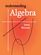Understanding Algebra (James W. Brennan)

An brief overview of pre-algebra and introductory algebra topics, written in a style meant to give you the basic facts in a way that is easy to understand, suitable for high-school Algebra I, or for anyone who wants to learn introductory algebra.

•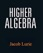Higher Algebra (Jacob Lurie)

This book is an informal and readable introduction to higher algebra at the post-calculus level with focus on infinite algebras;

•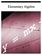Elementary Algebra (John Redden)

This textbook is the first part, written in a clear and concise manner, making no assumption of prior algebra experience. It carefully guides students from the basics to the more advanced techniques required to be successful in the next course.

•A First Course in Linear Algebra (Ken Kuttler)

The book presents an introduction to the fascinating subject of linear algebra. As the title suggests, this text is designed as a first course in linear algebra for students who have a reasonable understanding of basic algebra.

•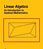Linear Algebra as an Introduction to Abstract Mathematics

This book aims to bridge the gap between the mainly computation-oriented lower division undergraduate classes and the abstract mathematics encountered in more advanced mathematics courses.

•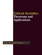Linear Algebra - Theorems and Applications (Hassan Abid Yasser)

This book contains selected topics in linear algebra, which represent the recent contributions in the most famous and widely problems. It includes a wide range of theorems and applications in different branches of linear algebra, etc.

•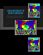Linear Mathematics In Infinite Dimensions (Ulrich H. Gerlach)

Focus on the mathematical framework that underlies linear systems arising in physics, engineering and applied mathematics - from the theory of linear transformation on finite dimensional vector space to the infinite dimensional vector spaces.

•Lectures on Linear Algebra and Matrices (G. Donald Allen)

This book avoids the traditional definition-theorem-proof format; instead a fresh approach introduces a variety of problems and examples all in a clear and informal style.

•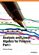Analysis and Linear Algebra for Finance (Patrick Roger)

This book presents the elements of analysis and linear algebra used in financial models and in microeconomics. Functions of one and several variables and matrices are developed as well as vector spaces, linear mappings and optimization methods, etc.

•Algebra and Trigonometry (Jay Abramson, et al)

This book provides a comprehensive and multi-layered exploration of algebraic principles. It is suitable for a typical introductory Algebra & Trigonometry course, and was developed to be used flexibly. The modular approach and the richness of content ensures that the book meets the needs of a variety of programs.

•Basic Category Theory (Tom Leinster)

Assuming little mathematical background, this short introduction to Category Theory is ideal for beginning graduate students or advanced undergraduates learning category theory for the first time.

•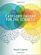Category Theory for the Sciences (David I. Spivak)

Using databases as an entry to Category Theory, this book explains category theory by examples, and shows that category theory can be useful outside of mathematics as a rigorous, flexible, and coherent modeling language throughout the sciences.

•Categories, Types, and Structures (Andrea Asperti, et al)

This book introduces Category Theory at a level appropriate for computer scientists and provides practical examples in the context of programming language design.

•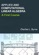Applied and Computational Linear Algebra: A First Course

This book is intended as a text for a graduate course that focuses on applications of linear algebra and on the algorithms used to solve the problems that arise in those applications.

•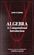Algebra: A Computational Introduction (John Scherk)

This book is a unique approach and presentation, the author demonstrates how software can be used as a problem-solving tool for algebra.

•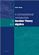A Computational Introduction to Number Theory and Algebra

This introductory book emphasises algorithms and applications, such as cryptography and error correcting codes, and is accessible to a broad audience.

•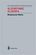Algorithmic Algebra (Bhubaneswar Mishra)

Anyone need to use algorithms in polynomials should have a copy of this! This is definitely one of the best! It covers almost everything anyone need in computation with systems of polynomial equations: Grobner Basis, Resultants, Characteristic Set, etc.

•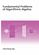Fundamental Problems of Algorithmic Algebra (Chee-Keng Yap)

This book provides a systematic and focused treatment of a collection of core problemsthe computational equivalents of the classical Fundamental Problem of Algebra and its derivatives.

•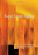Super Linear Algebra (W. B. V. Kandasamy, F. Smarandache)

Super Linear Algebras are built using super matrices. These new structures can be applied to all fields in which linear algebras are used. Super characteristic values exist only when the related super matrices are super square diagonal super matrices.

•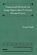Numerical Methods for Large Eigenvalue Problems (Yousef Saad)

This book is intended for researchers in applied mathematics and scientific computing as well as for practitioners interested in understanding the theory of numerical methods used for eigenvalue problems.

•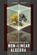Introduction to Non-linear Algebra (V. Dolotin and A. Morozov)

This unique text presents the new domain of consistent non-linear counterparts for all basic objects and tools of linear algebra, and develops an adequate calculus for solving non-linear algebraic and differential equations.

•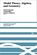Model Theory, Algebra, and Geometry (Deirdre Haskell, et al)

The book begins with an introduction to model theory. It then broadens into three components: pure model theory, geometry, and the model theory of fields.

•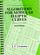Algorithms for Modular Elliptic Curves, 2nd Edition (J. E. Cremona)

This book presents a thorough treatment of many algorithms concerning the arithmetic of elliptic curves with remarks on computer implementation.

•Elementary Algebra (Denny Burzynski, Wade Ellis)

It is intended for students who (1) have no exposure to elementary algebra, (2) have previously had an unpleasant experience with elementary algebra, or (3) need to review algebraic concepts and techniques.

•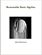Reasonable Basic Algebra for Students in Entering College

TThis book is a standalone version of part of From Arithmetic To Differential Calculus (A2DC), a course of study developed to allow a significantly higher percentage of students to complete Differential Calculus in three semesters.

•Orbital Integrals on Reductive Lie Groups and Their Algebras

This book is to present a complete course on global analysis topics and establish some orbital applications of the integration on topological groups and their algebras to harmonic analysis and induced representations in representation theory.

•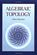Algebraic Topology (Allen Hatcher)

This introductory text is suitable for use in a course on the subject or for self-study, featuring broad coverage and a readable exposition, with many examples and exercises. The four main chapters present the basics: fundamental group and covering spaces, homology and cohomology, higher homotopy groups, and homotopy theory generally.

•Algebraic Combinatorics on Words (M. Lothaire)

This book is both a comprehensive introduction to the subject and a valuable reference source for researchers. An introductory chapter provides the reader with all the necessary background material.

•Algebraic Problems and Exercises for High School (Ion Goian, et al)

You will find algebra exercises and problems, grouped by chapters, intended for higher grades in high schools or middle schools of general education. Its purpose is to facilitate training in mathematics for students in all high school categories.

•Analysis and Linear Algebra for Finance (Patrick Roger)

This book presents the elements of analysis and linear algebra used in financial models and in microeconomics.

•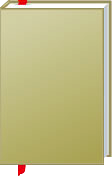Algebra, Abstract Algebra, and Linear Algebra

This is the previous page of Algebra, Abstract Algebra, and Linear Algebra, we are in the processing to convert all the books there to the new page. Please check this page daily!!!

Book CategoriesAll CategoriesRecent BooksIT Research LibraryMiscellaneous Books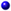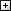Computer LanguagesComputer ScienceData Science/DatabasesElectronic EngineeringJava and Java EE (J2EE)Linux and UnixMathematicsMicrosoft and .NETMobile ComputingNetworking and CommunicationsSoftware EngineeringSpecial TopicsWeb Programming
Other Categories